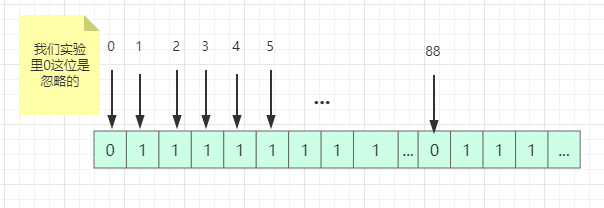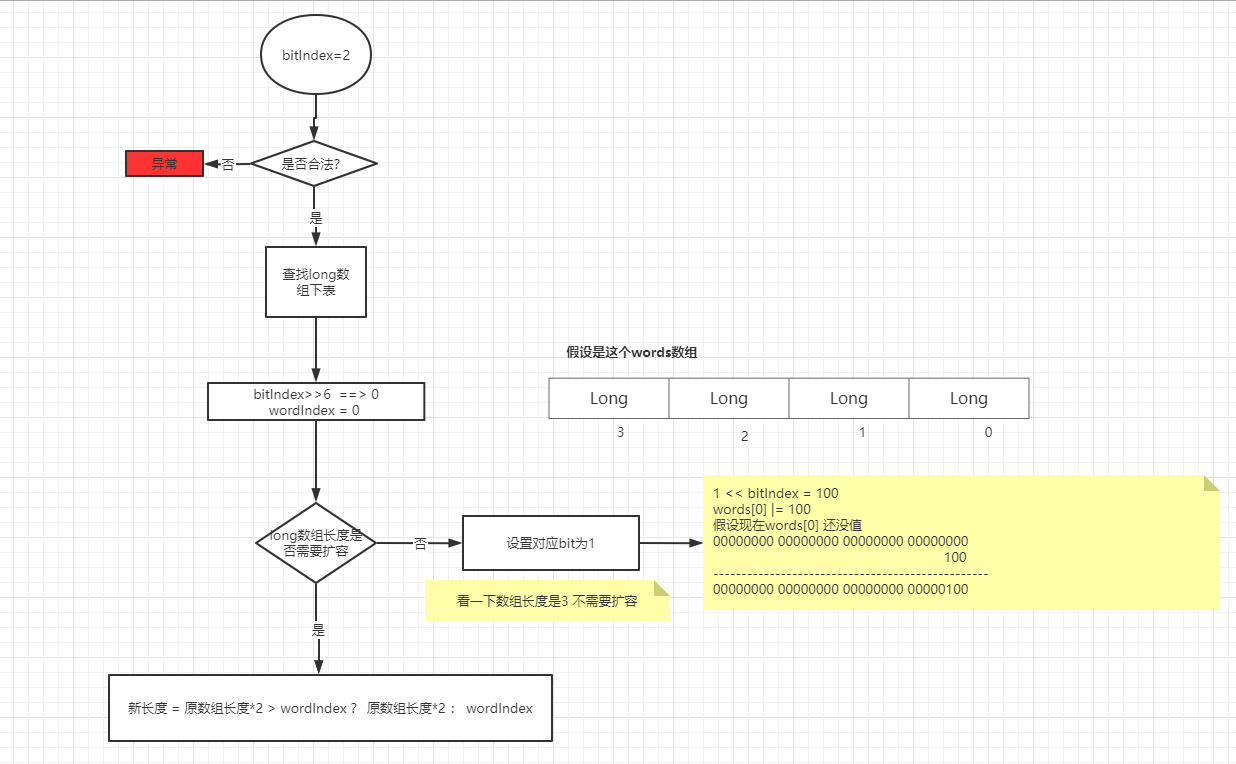# 面试题 ： 一个单调递增的数组 随机拿出一个数 你怎么找到这个数#### 一个单调递增的数组 被人随机拿出一个数 你怎么找到这个数

##### 1. 循环遍历 实现

/** * @author 木子的昼夜 */public class ConcurrnetTest {    public static void main(String[] args){        ConcurrnetTest test = new ConcurrnetTest();        Integer[] arr = test.get();        System.out.println(test.findByFor(arr));        // 或者是直接比较下标         System.out.println(test.findByFor02(arr));    }    // 遍历找数    private Integer findByFor(Integer[] arr) {        Integer res = null;        // 头尾处理  如果剔除的是1或者100        if(arr != 1) {            return 1;        }        if (arr[arr.length-1] != 100) {            return 100;        }        for (int i = 0; i < arr.length-1; i++) {            // 如果后一个不等于前一个+1 那就是被剔除了            if (arr[i]+1 != arr[i+1]) {                res = arr[i]+1;                break;            }        }        return res;    }       // 遍历找数    private Integer findByFor02(Integer[] arr) {        Integer res = null;        // 100如果被剔除 检测不到 需要特殊处理        if (arr[arr.length-1] != 100) {            return 100;        }        for (int i = 0; i < arr.length; i++) {            // 下标+1 不等于对应下表元素 就是有问题            // 0:1 1:2 2:3 ....            if (arr[i] != (i+1)) {                res = i+1;                break;            }        }        return res;    }    /**     * 获取 1 到 100  剔除88     * @return     */    public Integer[] get(){        List<Integer> list = new ArrayList<>();        for (int i = 1; i <= 100; i++) {            if (i != 88) {                list.add(i);            }        }        return list.toArray(new Integer);    }}

##### 2. BitSet 实现// 为什么101个  因为包含0   bit数组默认都是0 bit[] bits = new bit;// 遍历数组 数组中有1到100 剔除88for (int i = 0; i < arr.length; i++) {    // 设置对应的下标为1     bits[arr[i]] = 1;}

java 中 bit 数组不好实现 我们可以用 int 或者 long 的某一个二进制位表示

/** * @author 木子的昼夜 */public class ConcurrnetTest02 {    public static void main(String[] args){        ConcurrnetTest02 test = new ConcurrnetTest02();        Integer[] arr = test.get();        // 循环方式获取        System.out.println(test.findByBitSet(arr));    }    // 遍历找数    private Integer findByBitSet(Integer[] arr) {        // 从0 到 100        BitSet bitSet = new BitSet(101);        for (int i = 0; i <arr.length ; i++) {            bitSet.set(arr[i]);        }        // 从1 开始 到100  看哪个位是false 那就是哪个位没有值        // 这里的1 100 都可以写成参数 或者是配置  具体看自己实现        for (int i = 1; i <=100 ; i++) {            if (!bitSet.get(i)){                return i;            }        }        return null;    }    /**     * 获取 1 到 100  剔除88     * @return     */    public Integer[] get(){        List<Integer> list = new ArrayList<>();        for (int i = 1; i <= 100; i++) {            if (i != 88) {                list.add(i);            }        }        return list.toArray(new Integer);    }}

##### 3、简单了解一下 BitSet

3.1 构成

private long[] words; 

3.2 构造函数

// 指定默认大小public BitSet(int nbits) {        // 不能是负数  0也是可以的        if (nbits < 0)            throw new NegativeArraySizeException("nbits < 0: " + nbits);    // 初始化大小        initWords(nbits);        // 标记一下是否用户指定了大小         sizeIsSticky = true;}private void initWords(int nbits) {        // 算一下需要多少个64  也就是多少个long 然后初始化数据库         words = new long[wordIndex(nbits-1) + 1];}/*** ADDRESS_BITS_PER_WORD ： 6 */private static int wordIndex(int bitIndex) {    // >> 6 相当于 除以64  2^6=64    return bitIndex >> ADDRESS_BITS_PER_WORD;}

3.3 set 方法

 public void set(int bitIndex) {     // 非法bit位置     if (bitIndex < 0)         throw new IndexOutOfBoundsException("bitIndex < 0: " + bitIndex);     // 算一下这个位置对应long数组的哪个下标  bitIndex/64      int wordIndex = wordIndex(bitIndex);     // 检查是否需要扩容 需要的话直接扩容  默认扩展2*words.length      // 如果wordIndex>2*words.length 那就扩展到wordIndex大小     expandTo(wordIndex);   // 就是这个操作 设置了对应位置为1       // 1L << bitIndex 这句话就是把bitIndex转换为程序想要的bitindex     // 比如 ： 10 ==》 10000000000      // 然后 或运算  或就是只要一个为1 就为1       words[wordIndex] |= (1L << bitIndex); // Restores invariants     checkInvariants();}3.3 get 方法

 public boolean get(int bitIndex) {        // 非法判断        if (bitIndex < 0)            throw new IndexOutOfBoundsException("bitIndex < 0: " + bitIndex);    // 检测了个什么东西        checkInvariants();    // 获取下标        int wordIndex = wordIndex(bitIndex);        // 小于wordsInUse（在用数组最大下标） 这里用的&  对应下标是1 才返回true         return (wordIndex < wordsInUse)            && ((words[wordIndex] & (1L << bitIndex)) != 0);    }

3.4 其他方法

clear() 清空所有 设置所以位为falseclear(int bitIndex) 设置指定下标为false BitSet get(int fromIndex, int toIndex) 获取某个范围的值 返回BitSet set(int bitIndex, boolean value) 设置指定位置true或 false set(int fromIndex, int toIndex) 设置某个范围数据为true set(int fromIndex, int toIndex, boolean value) 设置某个范围数据为true或false clear(fromIndex, toIndex); 设置指定范围为falseand(BitSet set) 合并BitSet到自己  用的& 对应位置都为1 结果：就是1 or(BitSet set)合并BitSet到自己  用的| 对应位置只要有一个是1 结果：就是1 xor(BitSet set) 合并BitSet到自己  用的^  对应位置一个位1 一个为0 结果：就是1 andNot(BitSet set)   合并BitSet到自己  用的& ~ 原位置为1 set对应位置为0 结果：就是1## 评论